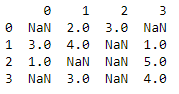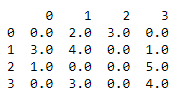Open In App

# Replace all the NaN values with Zero’s in a column of a Pandas dataframe

Replacing the NaN or the null values in  a dataframe can be easily performed using a single line DataFrame.fillna() and DataFrame.replace() method. We will discuss these methods along with an example demonstrating how to use it.

## DataFrame.fillna():

This method is used to fill null or null values with a specific value.

Syntax: DataFrame.fillna(self, value=None, method=None, axis=None, inplace=False, limit=None,                                  downcast=None)

Parameters: This method will take following parameters:

• value (scalar, dict, Series, or DataFrame):  Specify the value to use to fill null values.
• method([‘backfill’, ‘bfill’, ‘pad’, ‘ffill’, None], default None): Specify the method used to fill null values.
• axis (0 or ‘index’, 1 or ‘columns’): Specify the axis along which to fill missing values.
• inplace(bool, default False): If bool value is True, fill in-place which will modify any other views on this object.
• limit(int, default None): Specify the maximum number of consecutive NaN values to forward/backward fill.
• downcast(dict, default is None): A dict of item dtype of what to downcast if possible, or the string ‘infer’ which will try to downcast to an appropriate equal type (e.g. float64 to int64 if possible).

Returns: DataFrame or None. Object with null values filled or None if inplace=True.

Code: Create a Dataframe.

## Python3

 `# Import Pandas Library``import` `pandas as pd` `# Import Numpy Library``import` `numpy as np` `# Create a DataFrame``df ``=` `pd.DataFrame([[np.nan, ``2``, ``3``, np.nan],``                   ``[``3``, ``4``, np.nan, ``1``],``                   ``[``1``, np.nan, np.nan, ``5``],``                   ``[np.nan, ``3``, np.nan, ``4``]])` `# Show the DataFrame``print``(df)`

Output:Code: Replace all the NaN values with Zero’s

## Python3

 `# Filling null values``# with 0``df.fillna(value ``=` `0``,``          ``inplace ``=` `True``)` `# Show the DataFrame``print``(df)`

Output:## DataFrame.replace():

This method is used to replace null or null values with a specific value.

Syntax: DataFrame.replace(self, to_replace=None, value=None, inplace=False, limit=None, regex=False,                         method=’pad’)

Parameters: This method will take following parameters:

• to_replace(str, regex, list, dict, Series, int, float, None): Specify the values that will be replaced.
• value(scalar, dict, list, str, regex, default value is None): Specify the value to replace any values matching to_replace with.
• inplace(bool, default False): If a value is True, in place. Note: this will modify any other views on this object.
• limit(int, default None): Specify the maximum size gap to forward or backward fill.
• regex(bool or same types as to_replace, default False): If a value is True then to_replace must be a string. Alternatively, this could be a regular expression or a list,  dict, or array of regular expressions in which case to_replace must be None.
• method {‘pad’, ‘ffill’, ‘bfill’, None}: Specify the method to use when for replacement, when to_replace is a scalar, list or tuple and value is None.

Returns: DataFrame. Object after replacement.

Code: Create a Dataframe.

## Python3

 `# Import Pandas Library``import` `pandas as pd` `# Import Numpy Library``import` `numpy as np` `# Create a DataFrame``df ``=` `pd.DataFrame([[np.nan, ``2``, ``3``, np.nan],``                   ``[``3``, ``4``, np.nan, ``1``],``                   ``[``1``, np.nan, np.nan, ``5``],``                   ``[np.nan, ``3``, np.nan, ``4``]])` `# Show the DataFrame``print``(df)`

Output:Code: Replace all the NaN values with Zero’s

## Python3

 `# Filling null values with 0``df ``=` `df.replace(np.nan, ``0``)` `# Show the DataFrame``print``(df)`

Output: